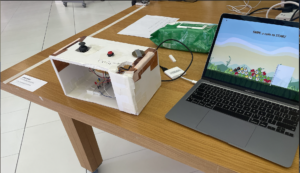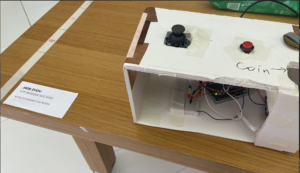# (Final Project) Toy Grabber Machine – Jade

VIDEOS / IMAGES

__________________PROCESS

__________________

How to play

First, the player should toss the coin into the coin pusher, which will start the game. Then the player uses the joystick to move the claw left or right, and the button to make the claw go down for the toys. I also added sound effects for different moves, so that it’s more interesting.

There is also a hidden prize which only three lucky persons today got it. Some people really wanted to get the prize and tried so hard at it — almost grabbed every toy.

Challenges

The coding part on Processing is quite challenging, and I spent quite some time on the física package so that I know how it works. One of the difficulties I encounter was the part where the claw goes down to grab, then drops it and finally returns.  It was such a headache to figure out how to make the claw know if it touches a toy or not. I solved this by setting another física object on the tip of the claw to detect physical collision. I also used many booleans to make this small animation.

The edge setting was also tricky. I have to make sure that the claw doesn’t go out of the screen or works weirdly. To avoid those rule-breaking people who wants to see what happens if the claw goes down in the drop box — I set it that the claw will just head back instead of going down. And if the claw doesn’t catch anything, as it touches the edge, it will automatically go back.

When it came to the Arduino part, the coding was much easier, and the physical setting wasn’t that complicated. The most hard part was the coin pusher, I had to glue the distance to the box really tight to make sure it measures the distance correctly.

But the joystick was really hard to control — the numbers it gave off were pretty random at first, but after I soldered it, I found the patterns of the numbers although it wasn’t really stable. Also, the cables and the distance sensor often fell off, and the port sometimes got disconnected.

Right before the IMA show, the port got disconnected and some wires fell off. Fortunately, during the entire show, everything worked perfectly and didn’t crash, which was amazing and surprising as well!

Conclusion

It was such an amazing journey of learning Processing and Arduino, and connecting them through serial communication to produce such a complex project. I could never expect to do something like this at the beginning of this semester.

I want to thank the professor for being supportive and giving great suggestions. Thank you for pointing out the problems with the machine  and helping me fix them before the show, so that they all worked great for the entire time. I feel sorry for not being able to let you play the perfect version 🙁

Thank you all for this fabulous course! Everyone’s projects are so creative and interesting! It was pleasant to work with everyone :))

CODE

__________________

Arduino:

```int joyX = A0;
int joyY = A1;
int button = 4;
int button_state;
const int trigPin = 7;
const int echoPin = 6;
int duration = 0;
int dist = 0;
int prize=0;

int red = 10;
int yellow = 9;
int blue = 8;

int posX;
int posY;

void setup() {
// put your setup code here, to run once:
Serial.begin(9600);
Serial.println("0,0,0,0");
pinMode(A0, INPUT);
pinMode(A1, INPUT);
pinMode(button, OUTPUT);
pinMode(trigPin , OUTPUT);
pinMode(echoPin , INPUT);
pinMode(red, OUTPUT);
pinMode(yellow , OUTPUT);
pinMode(blue , OUTPUT);
pinMode(2,INPUT);
digitalWrite(2,HIGH);
}

void loop() {
// put your main code here, to run repeatedly:
//  Serial.println(posY);
//  Serial.println(posX);

digitalWrite(red,prize);
digitalWrite(trigPin, LOW);
delayMicroseconds(2);
digitalWrite(trigPin, HIGH);
delayMicroseconds(2);
digitalWrite(trigPin, LOW);

duration = pulseIn(echoPin, HIGH);
dist = duration/58.2;
//  Serial.println(dist);

while (Serial.available()) {
prize = Serial.parseInt();
// move 0

if (posX >= 550) {
Serial.print(1);
} else {
Serial.print(0);
}
Serial.print(",");

// move 1
if (posX <= 360) {
Serial.print(1);
} else {
Serial.print(0);
}
Serial.print(",");

// grab 2
if (button_state == 0) {
Serial.print(0);
} else {
Serial.print(1);
}
Serial.print(",");

// coin pusher 3

if ( dist <= 5 )
{
Serial.println(1);
} else {
Serial.println(0);
}
digitalWrite(red,prize);
digitalWrite(yellow,prize);
digitalWrite(blue,prize);
}

}

}```

Processing:

```import fisica.*;
import processing.serial.*;
import processing.sound.*;

Serial myPort;

PImage claw;
PImage[][] claws;
PImage bird;
PImage[][] birds;
PImage bg;

boolean start = false;
boolean grab = false;
boolean get = false;
boolean drop = false;
boolean ret = false;
boolean prize = false;
boolean coin = false;
boolean right = false;
boolean left = false;

float speed;
float posX=20, posY=10;
FCircle[] bodies;

ArrayList<FBody> touching = new ArrayList<FBody>();
FCircle tip;
FBody prev_touch;

FWorld world;
int toy_num = 30;

SoundFile grab_sound;
SoundFile drop_sound;
SoundFile prize_sound;
SoundFile start_sound;

void setup() {
fullScreen();
size(750,600);

printArray(Serial.list());
String portname=Serial.list();
println(portname);
myPort = new Serial(this,portname,9600);
myPort.clear();
myPort.bufferUntil('\n');

//
bg.resize(displayWidth, displayHeight);
//bg.resize(750, 600);

translate((displayWidth-750)/2, (displayHeight-600)/2+100);

generate_claw();
Fisica.init(this);
world = new FWorld();
world.setEdges(0, 0, 750, 530, 255);
generate_birds();

tip = new FCircle(35);
tip.setFill(0);
tip.setStatic(true);
tip.setDrawable(false);

grab_sound = new SoundFile(this, "pick_up.wav");
drop_sound = new SoundFile(this, "drop.wav");
prize_sound = new SoundFile(this, "prize.wav");
start_sound = new SoundFile(this, "coin.wav");
}

void draw() {
//println(mouseX,mouseY);
background(bg);

translate((displayWidth-750)/2, (displayHeight-600)/2+100);
world.step();
world.draw();

// box
fill(255, 200);
noStroke();
rect(603, 250, 200, 280);
strokeWeight(3);
stroke(0);
line(603, 250, 605, 530);

if (coin) {
start_sound.play();
coin = false;
}

if (start){
initialize_claw();
} else {
fill(#A09B10);
PFont f = createFont("Dialog-48", 40);
textFont(f);
text("SWIPE a coin to START", 145, -100);
fill(#671235);
text("SWIPE a coin to START", 140, -100);
}
}

void initialize_claw() {
if (posX >= 750 - 80) {
posX = 750-80;
}

if (posX <= 20) {
posX = 20;
}

if (!grab) {
if (ret) {
if (posX > 20) {
image(claws, posX, 10);
posX -=3;
if (prize) {
fill(#901758);
text("CONGRATULATIONS!!!", 150, 100);
text("You are so LUCKY to get an AWARD", 80, 100);
}
} else {
ret = false;
world.remove(prev_touch);
prize = false;
}
} else {
image(claws, posX, 10);
if (right) {
posX += speed;
}

if (left) {
posX -= speed;
}
}
} else {
if (posX >= 550 && !get) {
grab = false;
get = false;
ret = true;
} else {

claw_down();
if (posY<0) {
posY+=3;
}
if (!drop) {
FBody toy = world.getBody(posX+60, posY+80);
if (get && posY < 20) {
image(claws, posX, posY);
if (posX <= 598) {
posX += 3;
toy.setPosition(posX+60, posY+80);
} else {
drop = true;
drop_sound.play();
toy.setStatic(false);
grab = false;
get = false;
drop = false;
ret = true;

// prize
int ram = (int)random(100);
if (ram <= 1) {
prize = true;
prize_sound.play();
}

}
}
}
}
}

}

void claw_down() {
FBody touch;
tip.setPosition(posX+40, posY+90);
if (posY < 500 && posY > 0) {
touching = tip.getTouching();
if (get) {
image(claws, posX, posY);
posY -= 3;
if (touching.size()>0) {
touch = touching.get(0);
prev_touch = touch;

touch.setStatic(true);
touch.setPosition(posX+60, posY+80);
}
} else{
image(claws, posX, posY);
if (touching.size()>0) {
get = true;
grab_sound.play();
} else {
posY += 3;
if (posY >= 500) {
grab = false;
get = false;
ret = true;
}
}
}
}
}

void generate_claw() {
claws = new PImage;
int w = claw.width / 5;
int h = claw.height / 8;

for (int y=0; y < 8; y++) {
for (int x=0; x< 5; x++) {
claws[y][x] = claw.get(x*w, y*h, w, h);
claws[y][x].resize(100,120);
}
}
}

void generate_birds() {
bodies = new FCircle;

birds = new PImage;
int w1 = bird.width / 3;
int h1 = bird.height / 2;

for (int y=0; y < 2; y++) {
for (int x=0; x< 3; x++) {
birds[y][x] = bird.get(x*w1, y*h1, w1, h1);
birds[y][x].resize(85, 85);
}
}

for (int i=0; i<toy_num; i++) {
b.setPosition(random(100, 500), height/2);
b.setStroke(0);
b.setStrokeWeight(2);
int random_x = int(random(0,2));
int random_y = int(random(0,3));
b.attachImage(birds[random_x][random_y]);
bodies[i] = b;
}

FBox edge = new FBox(10, 300);
edge.setPosition(600, 400);
edge.setStatic(true);
}

void mousePressed() {
// if button pressed, reset
world.clear();
grab = false;
ret = false;
get = false;
drop = false;
start = false;

bg.resize(displayWidth, displayHeight);
translate((displayWidth-750)/2, (displayHeight-600)/2+100);
generate_claw();
Fisica.init(this);
world = new FWorld();
world.setEdges(0, 0, 750, 530, 255);
generate_birds();

tip = new FCircle(35);
tip.setFill(0);
tip.setStatic(true);
tip.setDrawable(false);
posX = 20;
posY = 10;

initialize_claw();
}

void serialEvent(Serial myPort){
s=trim(s);
if (s!=null){
println(s);
int values[]=int(split(s,','));
if (values.length>=1){
if (values == 1) {
coin = true;
start = true;
}

if (values == 1 && start) {
left = false;
right = true;
} else {
right = false;
}

if (values == 1 && start) {
right = false;
left = true;
} else {
left = false;
}

if (values == 1 && start) {
grab = true;
}

}
}
myPort.write(int(prize)+"\n");

}```